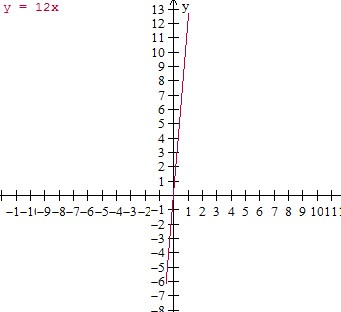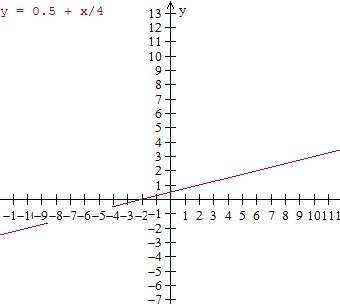# Graphing ordered pairs and writing an equation from a table of values in context

#### Excel Pivot Tables and Dashboard. Simple & Quick tutorial!

Most Popular

7 Lectures 58 mins

#### Learn HTML Tables

15 Lectures 59 mins

#### Excel Analytics - Data Analysis with Pivot-Tables and Charts

46 Lectures 2.5 hours

In this lesson, given a table of values, graphs are plotted from ordered pairs and equations are written.

Suppose we are given a table showing x and y values. The independent variable is represented by x and the dependent variable is represented by y and we have the ordered pairs of (x, y).

• From the ordered pairs, a graph is plotted

• From the table of values, an equation is found giving the relation between x and y.

Plot the ordered pairs described by the table. Write an equation relating x and y.

Input(x) Output(y)
0 0
1 12
2 24
3 36
4 48

### Solution

Step 1:

The equation of the ordered pairs of given table is

y = 12x

Step 2:

The plot of the ordered pairs of given table −Plot the ordered pairs described by the table. Write an equation relating x and y.

Input(x) Output(y)
0 0.5
4 1.5
8 2.5
12 3.5
16 4.5

### Solution

Step 1:

The equation of the ordered pairs of given table is

y = 0.5 + $\frac{x}{4}$

Step 2:

The plot of the ordered pairs of given table −